# Python Loops Tutorial with Examples

#### View more Tutorials: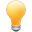Follow us on our fanpages to receive notifications every time there are new articles.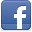Facebook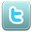Twitter

## 1- Overview of the loops in Python

In Python, statements are executed sequentially from top to bottom. However, when you want to execute a sequence of statements multiple times, you can use loop.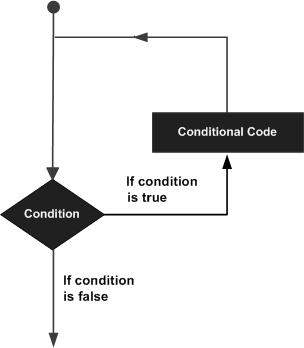Python provides you with 2 types of loops:
1. while loop
2. for loop
Statements are associated with the loop
• else
Statements can be used within a loop:
1. continue
2. break
3. pass
Control Statement Description

break

Terminates the loop statement.

continue

Causes the loop to skip the remainder of its body and immediately retest its condition prior to reiterating.

pass

The pass statement in the loop is simply a mark, prompting you to add some code in the future. It is a null statement (Do nothing).

## 2- while loop

The syntax of a while loop:
``````
while (condition) :
# Do something here
# ....
``````
Example:
whileLoopExample.py
``````
print("While loop example");
# Declare a variable, and assign value of 2.
x = 2;

# Condition is x < 10
# If x < 10 is true then run block
while (x < 10) :
print("Value of x = ", x);
x = x + 3;

# This statment is outside of while block.
print("Finish");
``````
Running the example:
``````
While loop example
Value of x = 2
Value of x = 5
Value of x = 8
Finish
``````

## 3- for loop with range

The simplest example of a for loop in Python is to use 'for' with 'range'. For example, the variable 'x' has a value that runs within the scope (3, 7) (x = 3, 4, 5, 6).
forLoopExample.py
``````
print("For loop example");
# for x = 3, 4, 5, 6
for x in range (3, 7) :
print("Value of x = ", x);
print("  x^2 = ", x * x);

# This statment is outside of for block.
print("End of example");
``````
Running the example:
``````
For loop example
Value of x = 3
x^2 = 9
Value of x = 4
x^2 = 16
Value of x = 5
x^2 = 25
Value of x = 6
x^2 = 36
End of example
``````

## 4- Use for loop and array

Using the for loop can help you traverse on the elements of the array.
forLoopExample3.py
``````
print("For loop example");
# Declare an array.
names =["Tom","Jerry", "Donald"];

for name in names:
print("Name = ", name);

print("End of example");
``````
Output:
``````
For loop example
Name = Tom
Name = Jerry
Name = Donald
End of example
``````
Traverse elements of the array through index:
forLoopExample3b.py
``````
print("For loop example");
# Declare an array.
names =["Tom","Jerry", "Donald"];

# len() function return length of array.
# index = 0,1,.. len-1
for index in range(len(names)):
print("Name = ", names[index] );

print("End of example");
``````

## 5- Use the break statement in the loop

break is a statement that may be located in a loop. This statement ends the loop unconditionally.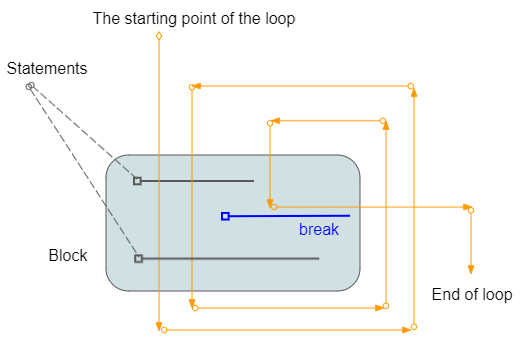loopBreakExample.py
``````
print("Break example");
# Declare a variable and assign value of 2.
x = 2;
while (x < 15) :
print("----------------------\n");
print("x = ", x);

# If x = 5 then exit the loop.
if (x == 5) :
break;
# Increase value of x by 1
x = x + 1;
print("x after + 1 = ", x);

print("End of example");
``````
Output:
``````
Beak example
----------------------
x = 2
x after + 1 = 3
----------------------
x = 3
x after + 1 = 4
----------------------
x = 4
x after + 1 = 5
----------------------
x = 5
End of example
``````

## 6- Use the continue statement in the loop

continue is a statement which may be located in a loop. When caught the continue statement, the program will ignore the command lines in block, below of continue and start of a new loop.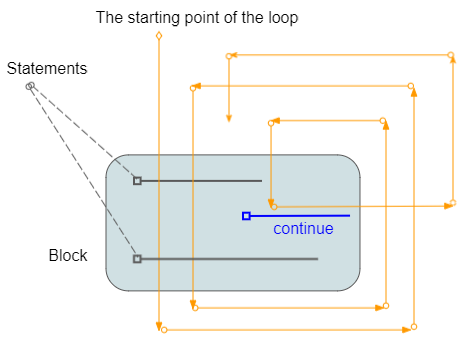loopContinueExample.py
``````
print("Continue example");

# Declare a variable and assign value of 2
x = 2

while (x < 7) :
print("----------------------\n")
print("x = ", x)

# % is used for calculating remainder
# If x is even, then ignore the command line below of continue
# and start new iteration.
if (x % 2 == 0) :
# Increase x by 1.
x = x + 1
continue
else :
# Increase x by 1.
x = x + 1
print("x after + 1 =", x)

print("End of example");
``````
Output:
``````
Continue example
----------------------
x = 2
----------------------
x = 3
x after + 1 = 4
----------------------
x = 4
----------------------
x = 5
x after + 1 = 6
----------------------
x = 6
End of example
``````

## 7- Use the pass statement in the loop

In Python programming, pass is a null statement. The difference between a comment and pass statement in Python is that, while the interpreter ignores a comment entirely, pass is not ignored.

However, nothing happens when pass is executed
The pass statement in the loop is simply a mark, prompting you to add some code in the future. It is a null command (Nothing).
loopPassExample.py
``````
number = 0

for number in range(5):
number = number + 1

if number == 3:
print(" do something here " , number)
pass

print(" >> " ,number )

print('Out of loop')
``````
Running the example:
``````
>> 1
>> 2
do somthing here 3
>> 3
>> 4
>> 5
Out of loop
``````
You can remove the pass statement in the example without changing anything.
loopPassExample.py (Remove pass statement)
``````
number = 0

for number in range(5):
number = number + 1

if number == 3:
print(" do something here " , number)
# pass (Remove pass)

print(" >> " ,number )

print('Out of loop')
``````

## 8- Use the 'else' statement with a loop

The else statement can be associated with a loop. The else statement is executed if the loop runs and ends in normal way, not broken by the break statement.
forLoopElseExample.py
``````
print("For loop example");

# for x = 3, 4, 5, 6
for x in range (3, 7) :
print("Value of x = ", x);
print("  x^2 = ", x * x);
else :
print("finish for loop")

# This statment is outside of for block.
print("End of example");
``````
Output:
``````
For loop example
Value of x = 3
x^2 = 9
Value of x = 4
x^2 = 16
Value of x = 5
x^2 = 25
Value of x = 6
x^2 = 36
finish for loop
End of example
``````
If the loop is stopped by the break statement, the else statement is associated with the loop will not be executed.
forLoopElseExample2.py
``````
print("For loop example");

# for x = 3, 4, 5, 6
for x in range (3, 7) :
print("Value of x = ", x);
if x == 5:
print("Break!")
break;
else :
# If the break statement has been called in the loop,
# this statement will not be executed.
print("This command will not be executed!")

# This statment is outside of for block.
print("End of example");
``````
Output:
``````
For loop example
Value of x = 3
Value of x = 4
Value of x = 5
Break!
End of example
``````

#### View more Tutorials:

Maybe you are interested

These are online courses outside the o7planning website that we introduced, which may include free or discounted courses.

•Python 3 For Beginner - Object-Oriented Programming
•Complete Python Web Course: Build 8 Python Web Apps
•Learning MongoDB - A Training Video From Infinite Skills
•JSP and Servlets - The Complete Course
•Master Computer Vision™ OpenCV4 in Python with Deep Learning
•Learning MongoDB-Volume 3
•C++ Intermediate: Build C++ Programs
•Complete Flutter development - build 14 ios and android apps
•Learn iPython: The Full Python IDE
•Learning iPython Notebook
•Python GUI : From A-to-Z With 2 Final Projects
•Python Fundamentals
•Python MySql From Scratch
•Basic Concepts of Web Development, HTTP and Java Servlets
•Python Programming Full Course (Basics,OOP,Modules,PyQt)
•Learning Path: Python GUI Projects
•Learning Path: Python: Design and Architect Python Apps
•Python 3000: Tactical SQL Quick-Start
•Python BeautifulSoup: Extract Web Data Beautifully
•Python 3000: Tactical File I/O
•Web Programming with Python
•Learning Path: Python Web Development
•Learn Programming in Python With the Power of Animation
•New to Python Automation..?Try Step by Step Python 4 Testers
•Python 2000: Beyond The Basics